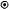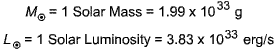Relation between Solar Luminosity and the Solar Constant The relationship between the solar constant and the solar luminosity is determined by simple geometry. Imagine a sphere the size of the Earth's orbit surrounding the Sun. The amount of solar energy passing through each square meter of the surface of this sphere is the solar constant, 1400 Watts per square meter. But since all energy emitted by the Sun must eventually pass through this spherical surface, the solar constant multipled by the total number of square meters on the surface of this sphere of radius 1 AU is the solar luminosity.

#### Solar Luminosity

A fundamental property of stars like the Sun is that they radiate enormous amounts of energy into space. Indeed, life on Earth would not exist but for that fact, since it is powered by the light from the Sun. We may measure the energy output of the Sun in several ways.
##### Total Radiated Energy
We may imagine placing the Sun in a sphere large enough to enclose it and determining how much energy radiated by the Sun passes through the sphere. The total amount of energy that passes through this sphere each second is called the solar luminosity. It is determined to be

Solar Luminosity ~ 4 x 1026 Watts

which is a huge amount of energy, equivalent to setting off a hundred-billion average-size hydrogen bombs every second.

##### The Solar Constant
We may measure the energy output of the Sun in another way. Imagine asking the question, "how much solar energy falls on each square meter of the upper atmosphere of the Earth each second?" This is related to the energy output of the Sun each second, but will be much less because the Earth is far away from the Sun and intercepts only a tiny fraction of the total energy that it emits. The amount of solar energy falling on a square meter of the Earth's upper atmosphere each second (integrated over all wavelengths) is called the solar constant. It is equal to about 1400 watts per square meter.

 Expressing Quantities in Solar Units It is often convenient to express some quantities in units of the corresponding quantity for the Sun. For example, mass and luminosity can be measured in units of solar masses and solar luminosities. It is common to denote the Sun by the symboland the solar values of quantities like masses are often indictated by using this symbol as a subscript. For example,are commonly used abbreviations for the unit of solar mass and the unit of solar luminosity.